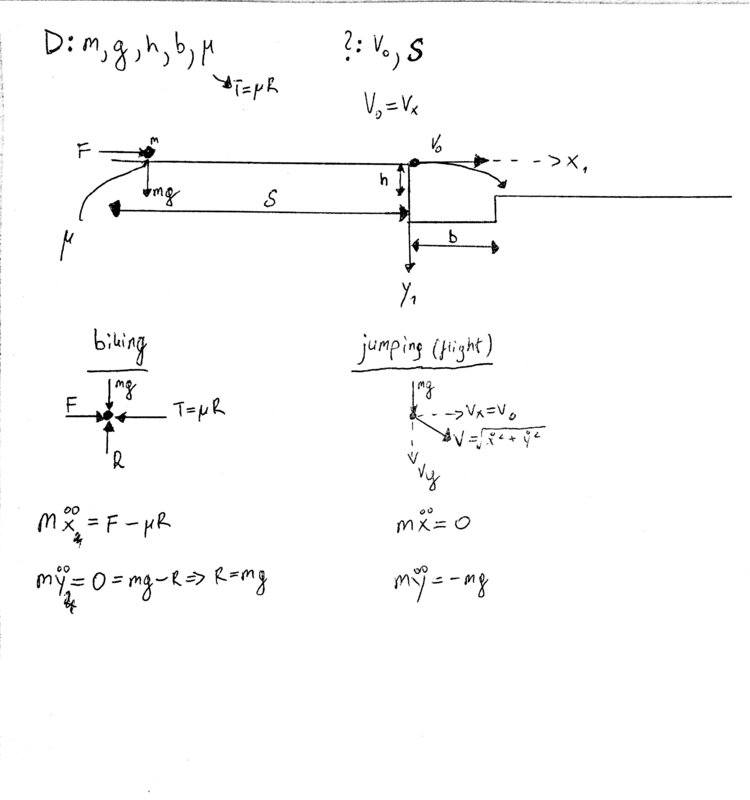# Particle Dynamics Problem (kinematics)

Thread moved from the technical forums, so no Template is shown
Summary: Mechanics problem related with Calculus (differential equations)Hi everyone, I would like some help in that task, if anyone would be willing to help :) Namely I have a problem from particle dynamics. "D:" means given info... so, D: m,g,h,b, miu. We're looking for v0 and S as given on the diagram. We need to work out differential equation problems for that task and need to have initial conditions clearly stated.

Underneath the diagram you can see the forces I have already assigned onto the particle body diagrams.

Thanks for all the help I can get from you guys! :)

Andrew Mason
Homework Helper
Hi aligator11. Welcome to PF!

It would help if you would describe what you are trying to do. It would be just a projectile motion problem if all you wanted to determine is the launch speed. If you want to determine S as well, we would have to know its initial speed. Can you give us the exact wording?

v0 (launch speed) is determined by the projectile motion part of the problem. So that is the first thing you have to determine. Express distance fallen as a function of time. That gives you the time required to jump the horizontal distance. Work out launch speed from that.

Assuming the initial speed is 0 (it starts at rest), express horizontal speed, v, as a function of acceleration and time. Then express distance in terms of acceleration and time.

AM

Yes the starting speed (1st initial speed) at the begining is 0 but we need to find v0, which is the initial speed just before the jump. So that would be problematic. I was trying to reorganize the data the best I could. In terms of the actual wording of the problem the only thing i got was the diagram I posted above, that's it. And of course the given data "D:..." and the components I have to find "?:...". Also we know that at the point where I have my coordinate system, v0=Vx so the horizontal component of velocity which we will probably use in regards with integration of the "jumping" part, system.

Andrew Mason